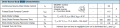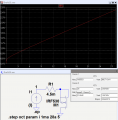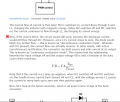# Body Diode Characteristics

Thread Starter

#### BramLabs

Joined Nov 21, 2013
98
Hello sir,

I want to ask, what are important parameters in the body diode characteristics of MOSFET. Let's say, i'm using IRF540 type of MOSFET.And i need to use the MOSFET as a SWITCH (using microcontroller), and i need the BODY DIODE to SYNCHRONIZE (means it will work as a normal diode at some MODE).

Let's say the current that goes through the diode, normally 4 Amps.

And the question is, what are important parameters on the datasheet i've attached above that need to be calculated so when i implement it, the MOSFET (with a normal HEATSINK) don't get TOO hot.

Thank you.

#### crutschow

Joined Mar 14, 2008
26,786
..................
Let's say the current that goes through the diode, normally 4 Amps.

And the question is, what are important parameters on the datasheet i've attached above that need to be calculated so when i implement it, the MOSFET (with a normal HEATSINK) don't get TOO hot.
It would be the value for Vsd at 4A, but that voltage is only given as 2.5V at a diode current of 28A, so what to do?
The simplest is to do a simulation of a similar MOSFET body diode.
(The best method, of course, is to physically measure an actual MOSFET).The closest model I had was for an IRF530 MOSFET.
A few LTspice simulations of that showed that an added 4.5mΩ resistor in series gave a drop of 2.5V @ 28A, the same as the IRF540 MOSFET body diode maximum voltage at the current.
This also showed a drop of about 0.9V @ 4A (below).

This gives a dissipation of about 3.6W when the diode is conducting 4A, which should be close enough to the actual value for engineering purposes.•BramLabs
Thread Starter

#### BramLabs

Joined Nov 21, 2013
98
It would be the value for Vsd at 4A, but that voltage is only given as 2.5V at a diode current of 28A, so what to do?
The simplest is to do a simulation of a similar MOSFET body diode.
(The best method, of course, is to physically measure an actual MOSFET).The closest model I had was for an IRF530 MOSFET.
A few LTspice simulations of that showed that an added 4.5mΩ resistor in series gave a drop of 2.5V @ 28A, the same as the IRF540 MOSFET body diode maximum voltage at the current.
This also showed a drop of about 0.9V @ 4A (below).

This gives a dissipation of about 3.6W when the diode is conducting 4A, which should be close enough to the actual value for engineering purposes.

View attachment 113225
Woah, you're awesome sir ! I need to learn A LOT from you. ahahahaha...

Thank you very much for the information. It really helps me !

Oh yeah, btw, an added 4.5 mOhms resistor in series, was it an example of internal Drain-Source Resistance of the MOSFET or the DIODE ?

Thank you so much.

#### crutschow

Joined Mar 14, 2008
26,786
....................
Oh yeah, btw, an added 4.5 mOhms resistor in series, was it an example of internal Drain-Source Resistance of the MOSFET or the DIODE ?
The body diode current does not go through the drain-source resistance path (since the MOSFET is OFF for this test with the gate shorted to the source) so the small resistance is added to the internal diode resistance just to make the simulation diode voltage match the spec sheet's value.

•BramLabs
Thread Starter

#### BramLabs

Joined Nov 21, 2013
98
The body diode current does not go through the drain-source resistance path (since the MOSFET is OFF for this test with the gate shorted to the source) so the small resistance is added to the internal diode resistance just to make the simulation diode voltage match the spec sheet's value.
Okay, thank you so much sir.
Btw, i want to implement bidirectional buck-boost converter, and i was wondering if it would be HOT if 4 amps current goes through it. ahahhah...Because last time, when i use P75NF75 mosfet from STmicroelectronics, the mosfet was really hot even with the heatsink (4 amps goes through the internal diode). ahahaah

#### dl324

Joined Mar 30, 2015
12,684
In a switching regulator, you'll get better efficiency using diodes with a faster reverse recovery time.

•BramLabs

#### crutschow

Joined Mar 14, 2008
26,786
Q2 is likely being used as a synchronous rectifier (it's turned ON when Q1 turns off to conduct current in the reverse direction and supply the current through L1. This works since a MOSFET conducts equally well in either direction when ON.).

That means the 4A will go through D2 only momentarily (the short time between Q1 turning off and Q2 turning on).

•BramLabs
Thread Starter

#### BramLabs

Joined Nov 21, 2013
98
In a switching regulator, you'll get better efficiency using diodes with a faster reverse recovery time.
So do i need another diode added to the circuit sir ?
Thank you btw for your reply.

Q2 is likely being used as a synchronous rectifier (it's turned ON when Q1 turns off to conduct current in the reverse direction and supply the current through L1. This works since a MOSFET conducts equally well in either direction when ON.).

That means the 4A will go through D2 only momentarily (the short time between Q1 turning off and Q2 turning on).
Yeah sir, that's why, if there's no Q2, the circuit will give Discontinuous Current Mode. But if there's a Q2 being in ON STATE, there will be reverse current through L1 when Q1 in OFF STATE, and it will be Continuous Current Mode, am i right ?

Thank you very much sir for your help.
I really appreciate it !

#### crutschow

Joined Mar 14, 2008
26,786
.....................
Yeah sir, that's why, if there's no Q2, the circuit will give Discontinuous Current Mode. But if there's a Q2 being in ON STATE, there will be reverse current through L1 when Q1 in OFF STATE, and it will be Continuous Current Mode, am i right ?
..................
I don't think so.
I don't understand what you mean by "if there's no Q2".Being in discontinuous mode or not is determined by the output current through the inductor, the value of the inductor, and the switching frequency.
It has nothing to do with the state of Q2 which will be switched the same in either mode.

•BramLabs
Thread Starter

#### BramLabs

Joined Nov 21, 2013
98
I don't think so.
I don't understand what you mean by "if there's no Q2".Being in discontinuous mode or not is determined by the output current through the inductor, the value of the inductor, and the switching frequency.
It has nothing to do with the state of Q2 which will be switched the same in either mode.
Oh really ?
Because i read on the internet ( http://electronics.stackexchange.co...uck-converter-chips-are-bidirectional-current ).
Is that correct what is written in the link ?Thank you so much sir.

Last edited:
Similar threads